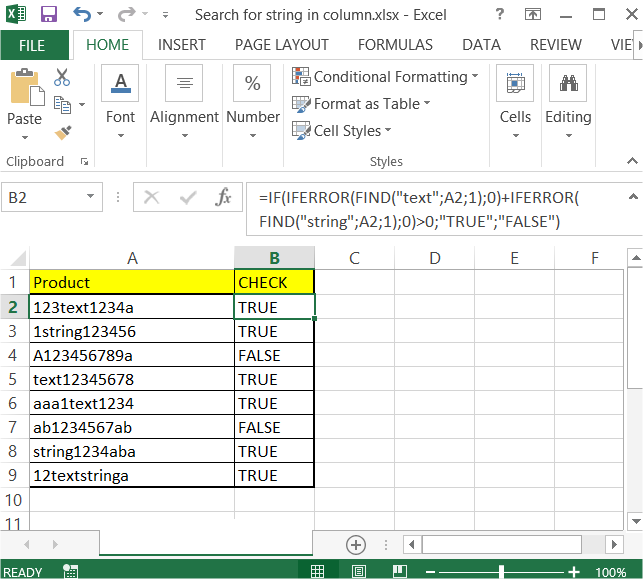### Search For String In Column

In this article you will teach yourself how to search for string in column. You may need this trick when you want to check if the cell contains string you need.

In this example you want to check if the cell contains either “text” or “string”.

In the data table below you see how data looks like.To check if “text” or “string” exist just use this formula:

=IF(IFERROR(FIND(“text”,A2,1),0)+IFERROR(FIND(“string”,A2,1),0)>0,”TRUE”,”FALSE”)This formula will display TRUE or FALSE in B column. You can use conditional formatting if you want.

### Extracting first/nth/last word from the string

Let’s see now how to extract the first, the nth or the last word from the string.

Suppose you have some string in A2 cell. You want to extract some part of this string. To do this you need to use formulas below.

To extract the first word from the string just use this formula:

=IFERROR(LEFT(A2,FIND(” “,A2)-1),””)

You may also extract the first word from the string with a leading space:

=IFERROR(LEFT(A2,FIND(” “,A2)),””)

This is a formula which let you extract the nth word. You string is A1 cell and the number of occurrence is in B1.

=TRIM(RIGHT(SUBSTITUTE(LEFT(TRIM(A2),FIND(“^^”,SUBSTITUTE(TRIM(A2)&” “,” “,”^^”,B2))-1),” “,REPT(” “,100)),100))

And finally the last word you can extract with below Excel formula:

=IF(ISERR(FIND(” “,A2)),””,RIGHT(A2,LEN(A2)-FIND(“*”,SUBSTITUTE(A2,” “,”*”,LEN(A2)-LEN(SUBSTITUTE(A2,” “,””))))))

```Further reading: